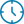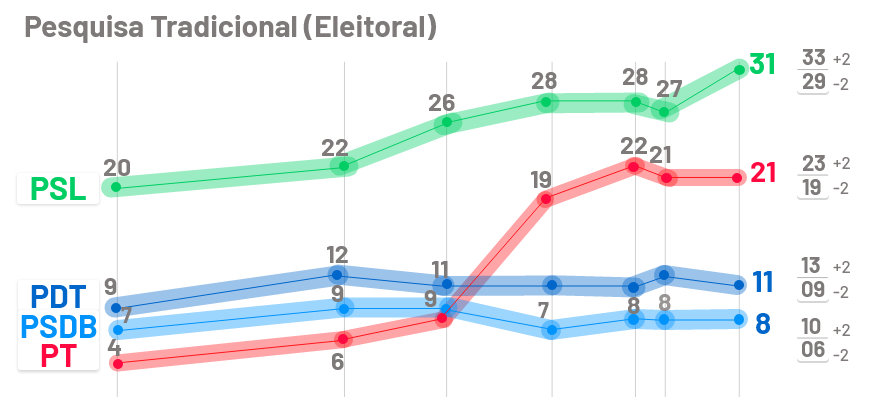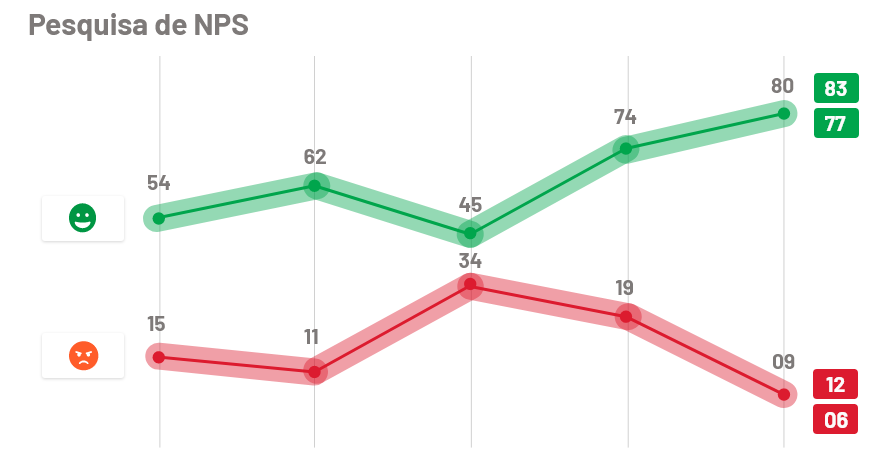# Sample error for satisfaction surveys## CALL US

##### Categories4 min

As in election polls, most quantitative surveys use the sample error formula to determine their level of accuracy.

However, as exemplified in the text of the link above, this formula works to evaluate competing results, that is, in an election poll, you will assess the chances of victory among candidates A, B, C e D.In the example in the figure, above we have the sampling error of ± 2% associated with each candidate.

For satisfaction surveys like CSAT, CES, Likes and Dislikes among others with multiple choice, this methodology works and is the most suitable.

### Why this method doesn't work for NPS?

Paralleling electoral research, let's assume that the Promoter is a candidate and Detrator is the other.In the example in the figure above we have the sample error of ± 3% associated with each type of customer.

Unlike the direct-reading multiple-choice surveys described above (CSAT, CES, Likes & Multiple choice dislikes), o NPS (Net Promoter Score) consists of a subtraction between the types of customers (Promoters and Detractors).:

NPS = % Promoters – % Detractors

Thus, by subtracting the Detractors from the Promoters, each with a margin of error of ± 3%, we would be adding the error of the two categories of customers and obtaining ± 6% of sampling error.

### And then? What is the best way for NPS?

Standard Error must be calculated first (I KNOW) or Standard error, as shown to follow:

Soon after, the confidence interval or error margin is obtained., in the following way:

NPS ± 1,96 * I KNOW

Being 1,96 the value referring to a confidence level of 95%.

### To facilitate understanding we exemplify for you:

Let's say you did a survey that you received 10.000 answers, in which 6000 (60%) were promoters, 2000 (20%) were neutral and 2000 (20%) were detractors and want to calculate the NPS error.

NPS = 60 – 20 = 40

First step: Following our example above, let's calculate the Standard Error (I KNOW), this is, the Standard Error of the search. Obtaining the following values:

Promoter = 0,6
Neutral = 0,2
Detrator = 0,2
Standard Error (I KNOW) = 0,8%

Second step: Knowing the Standard Error, identified in the first step, we will determine the confidence interval (Margin of error) do NPS, considering a reliability of 95%:

Margin of error: 1,96 * 0,8 = 1,6

Going back to our example, the NPS confidence interval would now be:

NPS = 40 with ± error range 1,6

That is, when conducting research with different samples from your customers, your NPS will be among 38,4 and 41,6 in 95% of the times.

### But what if I want to improve my error margin in NPS? How do I do? I need lots of reviews?

We have prepared for you a summary so you don't worry about it anymore! Check below the amount of answers you need to have the desired margin of error on your NPS!

As our goal with this text is to reduce your effort, we make this available Sample Error Calculator and Sample Size for satisfaction surveys, where you can apply to each of the methodologies.

See how easy it is? If you have any questions about some of the steps or want to go deeper into the theory, we made available the complete study on the Sample error applied to NPS.